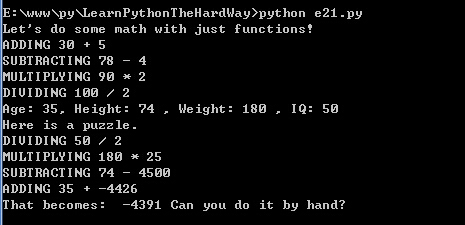## Learn Python The Hard Way（21） 原

You have been using the = character to name variables and set them to numbers or strings. We're now going to blow your mind again by showing you how to use = and a new Python word return to set variables to be a value from a function.There will be one thing to pay close attention to, but first type this in:

``````#!/usr/bin/env python
# -*- coding: utf-8 -*-

def add(a, b):
print "ADDING %d + %d" % (a, b)
return a + b

def subtract(a, b):
print "SUBTRACTING %d - %d " % (a, b)
return a - b

def multiply(a, b):
print "MULTIPLYING %d * %d " % (a, b)
return a * b

def divide(a, b):
print "DIVIDING %d / %d " % (a, b)
return a / b

print "Let's do some math with just functions!"

age = add (30 , 5)
height = subtract(78,4)
weight = multiply(90, 2)
iq = divide(100, 2)
print "Age: %d, Height: %d , Weight: %d , IQ: %d " % (age, height,weight , iq)
# A puzzle for the extra credit, type it in anyway.
print "Here is a puzzle."
what = add (age, subtract(height, multiply(weight, divide(iq,2))))
print "That becomes: ", what, "Can you do it by hand?"``````

result:We are now doing our own math functions for add, subtract, multiply, and divide. The important thing to notice is the last line where we say return a + b (in add ).

Extra Credit

1. If you aren't really sure what return does, try writing a few of your own functions and have them return               some values. You can return anything that you can put to the right of an =.

2. At the end of the script is a puzzle. I'm taking the return value of one function, and using it as the                       argument of another function. I'm doing this in a chain so that I'm kind of creating a formula using the               functions. It looks really weird, but if you run the script you can see the results. What you should do is try           to figure out the normal formula that would recreate this same set of operations.

3. Once you have the formula worked out for the puzzle, get in there and see what happens when you                   modify the parts of the functions. Try to change it on purpose to make another value.

4. Finally, do the inverse. Write out a simple formula and use the functions in the same way to calculate it.

© 著作权归作者所有### 嚣张乾坤

25 本免费的 Python 编程电子书

2013/06/14
2.4K
6

2013/10/13
488
2

2015/06/29
440
2

scroll
2013/01/12
81K
17

CSDN资讯
03/14
0
0

Spring Boot + Mybatis-Plus 集成与使用（二）

7
0

@[TOC](用最通俗的方法讲spring [一] ──── AOP) 写这个系列的目的(可以跳过不看) 自己写这个系列的目的,是因为自己是个比较笨的人,我曾一度怀疑自己的智商不适合干编程这个行业.因为在我...

7
0
Flutter系列之在 macOS 上安装和配置 Flutter 开发环境

6
0
OSChina 周六乱弹 —— 早上儿子问我他是怎么来的

Osc乱弹歌单（2019）请戳（这里） 【今日歌曲】 @凉小生 ：#今日歌曲推荐# 少点戾气，愿你和这个世界温柔以待。中岛美嘉的单曲《僕が死のうと思ったのは (曾经我也想过一了百了)》 《僕が死の...

2.7K
16
Excption与Error包结构，OOM 你遇到过哪些情况，SOF 你遇到过哪些情况

Throwable 是 Java 中所有错误与异常的超类，Throwable 包含两个子类，Error 与 Exception 。用于指示发生了异常情况。 Java 抛出的 Throwable 可以分成三种类型。 被检查异常（checked Exc...

Garphy

42
0